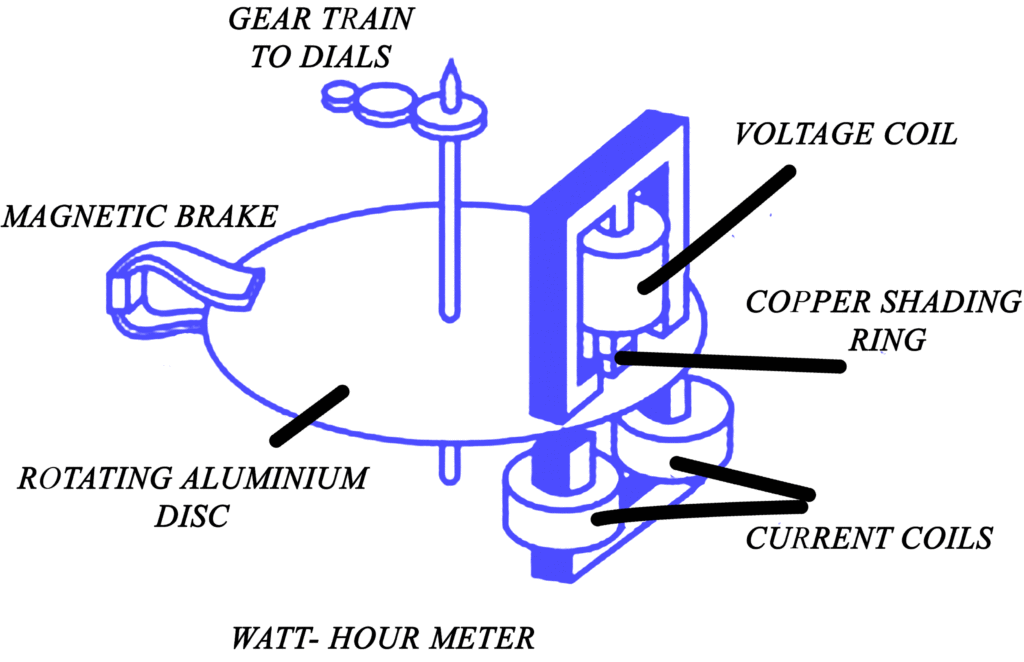Home / Instrumentation / AC Energy Meter Working Principle

# AC Energy Meter Working Principle

Want create site? Find Free WordPress Themes and plugins.

Energy is the product of power and time and is measured in watt-seconds or Joule. Since the voltage and current in a DC circuit are constant values, the energy is easily computed from a measurement of power and time,

$W=Pt~~~~~~~~~~Joule$

Where W is in watt-seconds or Joules, P in watts and t in seconds. The watt second is a too small unit and hence the larger unit kilowatt-hour (KWH) is preferred.

If the current and voltage are not constant, one measures energy directly by the use of a watthour meter. Both AC and DC watt-hour meters are available, both types are summing or integrating types. The basic assembly of an AC induction watthour meter is shown in Fig.The AC induction watthour meter has voltage and current coils, but unlike the wattmeter, all the coils are stationary. The voltage coil is connected to the source lines, whereas the current coil is connected in series with the load. The combination of stationary coils is called the stator.

You May Also Read: Power Measurement In a Three-Phase System using Wattmeter

A disk or rotor mounted on a shaft receives a torque through an electromagnetic induction whenever the two sets of coils are energized. The rotor is mechanically connected to a meter register via a gear train. A register provides a record of a number of rotor shaft revolutions and is calibrated in kilowatt-hours. A constant of proportionality, the watthour constant, Kh, is the number of watthours corresponding to one disk revolution. Thus the energy in watt-hours is

$W={{K}_{h}}*disk~revolutions$

And in kilowatt-hours;

$W=\frac{{{K}_{h}}*disk~revolutions}{1000}$

Did you find apk for android? You can find new Free Android Games and apps.